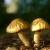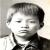## 针对avr atmega328p，利用硬件定时器构建实时多任务的一种设计方法

pwm的周期是20ms，0-180度的脉宽为0.5ms - 2.5ms，带负载下舵机最快输出转速大于60度／秒，（正常舵机无负载下，可以有60度／0.2sec的速度）。

0.5 ＋ 45 ／180 * (2.5 - 0.5) = 1ms

20ms * 1000 / 0.5 = 40000
1ms * 1000 / 0.5 = 2000

```TCNT1 = (1ul << 16) - 40000ul
OCR1A = (1ul << 16) - 40000ul + 2000ul```

`TCNT1 = (1ul << 16) - 40000ul`

```#define SETA_PWMWIDTH(angle) do{\
OCR1A = (1ul << 16) - 40000ul + ((angle) * 2000 * 2/ 180 + 500 * 2);\
}while (0)
// 1000 * 2 -> 1ms * 1000 / 0.5
// 2000 * 2 -> 2ms * 1000 / 0.5 ```

```#define SERVO_2_ANGLE(angle) do{\
uint8_t _tmpangle = max(180,min((angle),0));\
SETA_PWMWIDTH(_tmpangle);\
}while(0)```

```#define SETB_PWMWIDTH(angle) do{\
OCR1B = (1ul << 16) - 40000ul + ((angle) * 2000 * 2/ 180 + 500 * 2);\
}while (0)
#define SERVOB_2_ANGLE(angle) do{\
uint8_t _tmpangle = min(180,max((angle),0));\
SETB_PWMWIDTH(_tmpangle);\
}while(0)```

1、按照8分频设置计数器
2、设置一个数组，每个单元里存放对应舵机脉宽持续的时间（换算成次数），并排序
3、选择数组中最小值作为当前的OCR1A的值，使得到这个点触发一次比较中断，处理对应引脚拉低。
4、比较中断中，将数组中下一个值给予OCR1A，退出中断。

(1ul << 16) - 40000ul ＋ 3000

1、相邻两个数值差大于等于32

2、修改后的偏移量不会超过原先位置的16，也即不会形成误差累计。

```uint8_t i;
registor uint16_t m,M,Mo;
for (i = 0 ; i < n - 1 ; i++){
m = a[i];M = a[i+1];
if (m + 32 < M){
continue;
}
if (m + 16 >= M){
a[i+1] = m;
continue;
}
M = a[i + 1] = m + 32;
for (j = i + 2 ;j < n ;j++){
if (a[j] <= M){
a[j] = M;
}else{
break;
}
}
i = j - 2;
}```

(注意上面代码没有实测，本帖子所有代码都没实测过，只讲道理，不抓bug，哈)

1、如果当前点和后续点之间的差值大于32，则不考虑他们的差异（此时保持原有精度）
2、如果当前两个点的差值小于16，进行修正后者等于前者，其认为两个点是相同的比较值，此时和第一种调整方法的效果相对等同。
3、如果当前两个点的差值介入16到32之间，则将后一个点的比较值增加，保证两点之间至少32个计数间隔。但此时可能会导致后续的一些点小于了调整后的点，例如
1，31，32

1、上述8分频16位计数器的最大周期， 65536 * 0.5us = 32768us = 32.768ms，如果遇到低频事件，例如20Hz，每个周期50ms，上述计数器就玩不转了。可能增加如下代码也行：

```uint8_t flag = 0;

...
if (flag == 0){

}else{
//do something ...
}
flag = !flag;```

2、中断发生，中断使能被清，中断响应函数结束是reti，会重新设置中断使能。简单说，响应过程中不会形成嵌套。这是好事，不混乱，也可能是坏事，会漏事情。

1、舵机本身保持一个角度，需要20ms周期，在0.5-2.5之间存在高电平的脉宽输出事件。
2、我们希望舵机按照一个速度转到另一个角度，则可能需要将转角差值等分（至少等分吧）在多个周期中，例如现在希望按照每秒10度的速度运转2秒，则我们构造一个10Hz的角度修正事件，每次设置一次舵机的目标角度，也即增加1度。
3、无论是遥控，或者红外巡线，或者视频识别，总要定期的获取当前执行单元对应的执行目标－也即，“执行任务”检测事件。

```#define FUNC_MAX 8
uint8_t flag ;
uint8_t func_count[FUNC_MAX];
uint8_t func_cycle[FUNC_MAX];//1 cycle is 1ms
uint16_t millis = 0;
uint16_t last_millis = 0;
typedef uint8_t(*_MODULE_FUNC)(void);
_MODULE_FUNC pfunc[FUNC_MAX] = {.....};

void xxxx_interrupt(...){
uint8_t i;
uint8_t delta;
millis++;
delta = millis - last_millis;
if (flag){
return;
}
flag = 1;
for (i = 0 ; i < FUNC_MAX ;i++){
if (func_count[i] > delta){
func_count[i] -= delta;
}else{

func_count[i] = func_cycle[i];
sei();
pfunc[i]();
cli();
}
}

cli();
last_millis = millis;
flag = 0;
}```

```while (1){
xxx();
yyy();
delay();
}```

```while (1){
swtich (status){
case :
....
case :
...
default:
delay(xx);
}
}```

```void delay(uint8_t xx){
while (xx--){
for (i = 0 ; i < 2048; i++){
nop();
nop();
nop();
nop();
nop();
nop();
}
}
}```

“啊这样我怎么用delay控制led灯每秒闪两次啊，怎么能大差不差呢？”

00#### 引用来自“管梨员”的评论